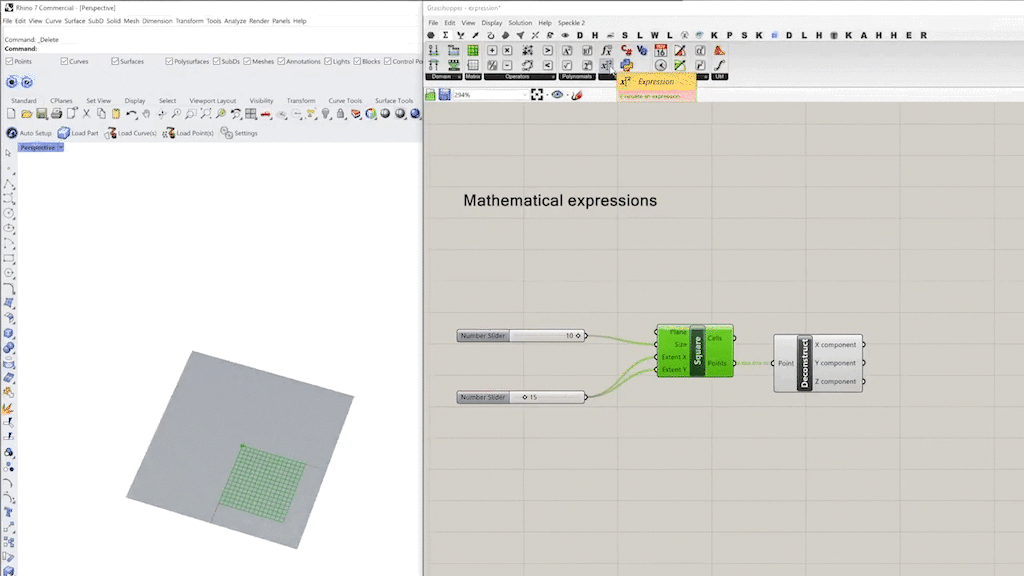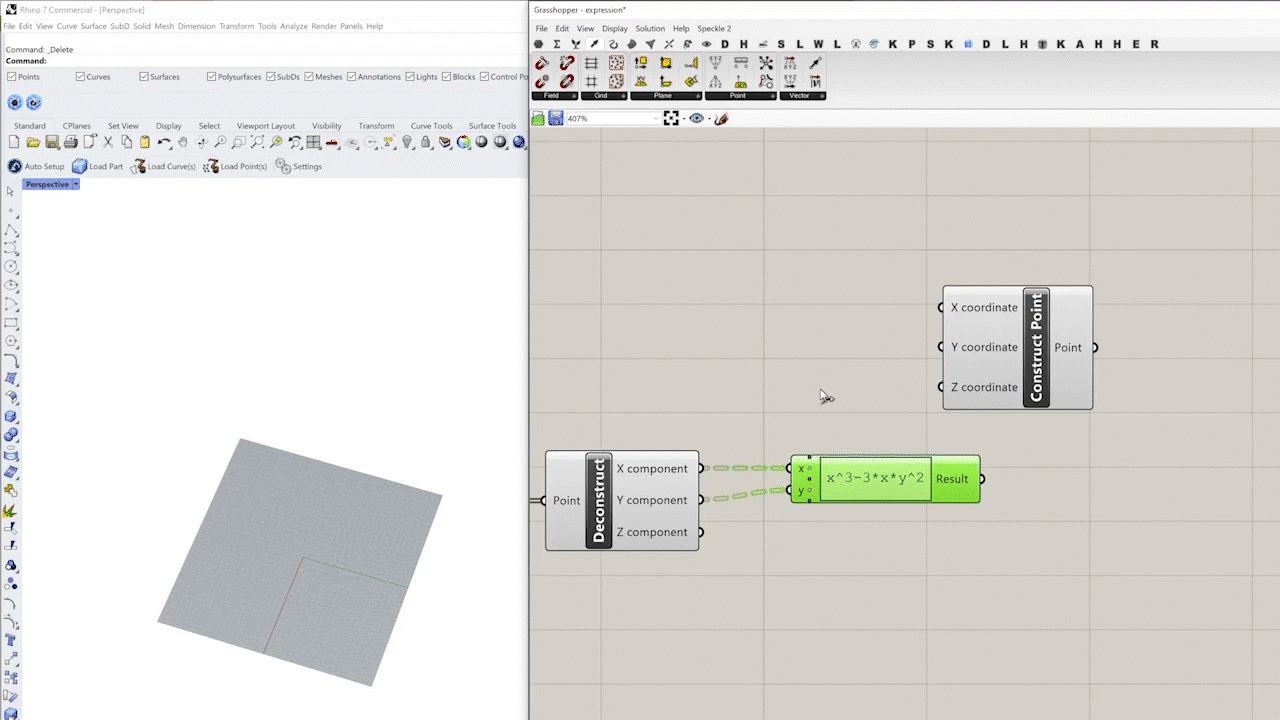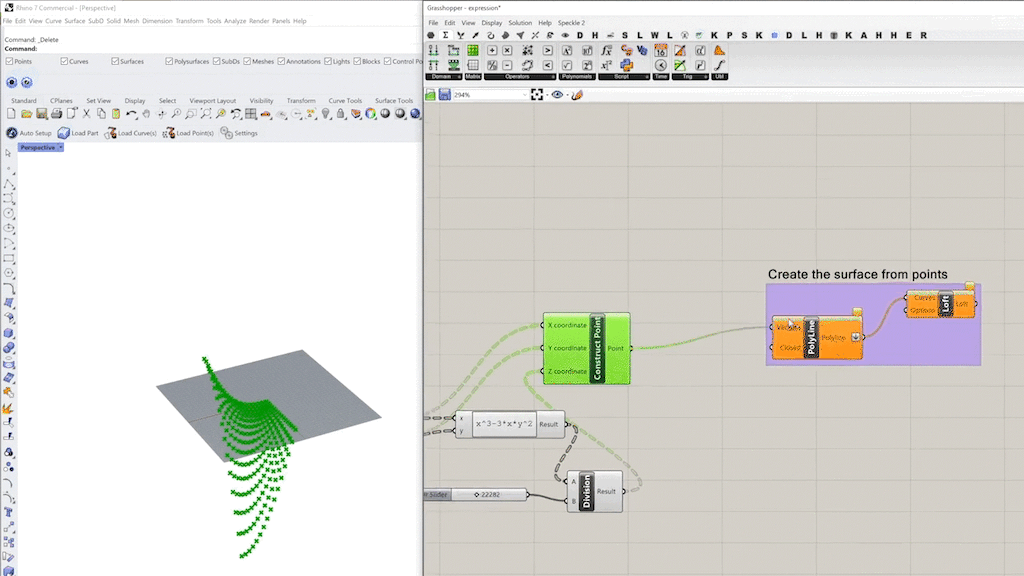top of page
Search

# 018 – Mathematical expressions in Grasshopper

## Mathematical Expressions

We can solve mathematical formulas and output numerical data by using an expression component. In this example, we will create a saddle surface which is a mathematical surface using the expression component.

## Steps:

Step 1: First you will need x,y,z coordinates to create a surface. We can use the 'grid' component to create a grid of points.Step 2: From the grid we use the 'deconstruct points' component to extract the

x,y,z coordinates.Step 3: Now we need to use the 'expression' component. The simple Cartesian equation for the saddle surface is z=x(x^2-3y^2). But for simplicity we will write it as z=x^3-3*x*y^2.Note: The input variables should be the same as in the formula. In this case, x and y.

Step 4: Now using the new z variable from the formula, we can create the new point set with the 'construct point' component.Note: Since we are dealing with a large number derived from the formula, we need to use the 'divide' component to make z smaller.

And this is the result: